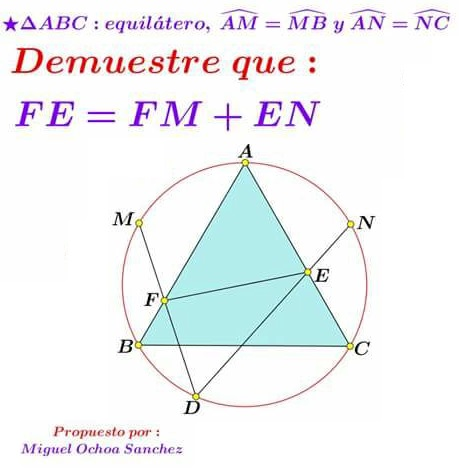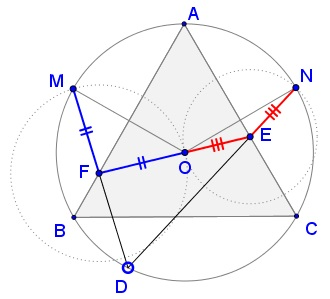# One More Property of Equilateral Triangles

### Source### Problem### Solution

Let $O\,$ be the center of $(ABC).\,$ Then, not only $OM\perp AB\,$ and $ON\perp AC,\,$ but $OM\,$ and $ON\,$ are divided in half by the points of intersection.This makes triangles $OFM\,$ and $OEN\,$ isosceles so that $FM=FO\,$ and $EN=NO.\,$ Suffice it to show that $O\in EF.$ This is achieved by angle chasing.

\displaystyle \begin{align} \angle MDN&=60^{\circ},\\ \angle AFM&=\frac{1}{2}(\overset{\frown}{AM}+\overset{\frown}{BD}),\\ \angle AEN&=\frac{1}{2}(\overset{\frown}{AN}+\overset{\frown}{CD}),\\ \angle AFM+\angle AEN&=\frac{1}{2}(2\cdot 60^{\circ}+(\overset{\frown}{BD}+\overset{\frown}{CD})),\\ &=120^{\circ},\\ \angle OFM+\angle OEN&=2(\angle AFM+\angle AEN)=240^{\circ},\\ \angle OFD+\angle OED&=360^{\circ}-2(\angle AFM+\angle AEN)=120^{\circ}. \end{align}

It follows that $\angle OFD+\angle OED+\angle EDF=180^{\circ},\,$ because $\angle EDF=\angle MDN,\,$ which makes $EOF\,$ a straight line.

### Acknowledgment

The above problem was kindly posted by Miguel Ochoa Sanchez at the CutTheKnotMath facebook page.

• Angle Trisectors on Circumcircle
• Equilateral Triangles On Sides of a Parallelogram
• Pompeiu's Theorem
• Pairs of Areas in Equilateral Triangle
• The Eutrigon Theorem
• Equilateral Triangle in Equilateral Triangle
• Seven Problems in Equilateral Triangle
• Spiral Similarity Leads to Equilateral Triangle
• Parallelogram and Four Equilateral Triangles
• A Pedal Property in Equilateral Triangle
• Miguel Ochoa's van Schooten Like Theorem
• Two Conditions for a Triangle to Be Equilateral
• Incircle in Equilateral Triangle
• When Is Triangle Equilateral: Marian Dinca's Criterion
• Barycenter of Cevian Triangle
• Excircle in Equilateral Triangle
• Converse Construction in Pompeiu's Theorem
• Wonderful Trigonometry In Equilateral Triangle
• 60o Angle And Importance of Being The Other End of a Diameter
• Van Khea's Quickie
• Equilateral Triangle from Three Centroids
•